Computational & Technology Resources
an online resource for computational,
engineering & technology publications
Civil-Comp Proceedings
ISSN 1759-3433
CCP: 75
PROCEEDINGS OF THE SIXTH INTERNATIONAL CONFERENCE ON COMPUTATIONAL STRUCTURES TECHNOLOGY
Edited by: B.H.V. Topping and Z. Bittnar
Paper 36

Triply Coupled Vibrations of Thin-Walled Open Cross-Section Beams including Shear Effect

A. Arpaci, E. Bozdag and E. Sunbuloglu

Department of Mechanical Engineering, Istanbul Technical University, Turkey

Full Bibliographic Reference for this paper
A. Arpaci, E. Bozdag, E. Sunbuloglu, "Triply Coupled Vibrations of Thin-Walled Open Cross-Section Beams including Shear Effect", in B.H.V. Topping, Z. Bittnar, (Editors), "Proceedings of the Sixth International Conference on Computational Structures Technology", Civil-Comp Press, Stirlingshire, UK, Paper 36, 2002. doi:10.4203/ccp.75.36
Keywords: coupled vibration, thin-walled beam, shear effect.

Summary
Beams of thin-walled open cross-sections are widely used in structural design. In general, the centroid and the shear centre do not coincide; hence the flexural and the torsional vibrations are coupled. The case that the flexural vibrations in one direction are coupled with the torsional vibrations is extensively studied by many researchers. But, the case that the flexural vibrations in two mutually perpendicular directions and the torsional vibrations are all coupled is not sufficiently dealt with. Gere and Lin  have determined the triply coupled free vibration characteristics of uniform, open cross-section channels with simply supported ends by employing the Rayleigh - Ritz method. Yaman  has formulated the mathematical model by using the wave propagation approach and has presented the wave numbers for undamped and structurally damped channels. Arpaci et al.  have studied effect of rotary inertia on triply coupled vibrations of thin-walled beams. To the authors' knowledge, no work considering the shear effect on triply coupled vibrations of such beams appears in literature. The objective of the present study is to investigate the effect of shear on triply coupled vibrations of beams with thin-walled open cross-sections having no axis of symmetry. The effects of cross-sectional warping are also included.

#### Equations of Motion

In Figure 36.1 is shown a typical cross-section of no axial symmetry where the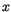and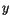axes are taken through the shear centre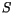and parallel to the principal centroidal axes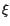and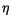. The equations of motion may be derived by substituting inertia forces into the equations of static equilibrium as follows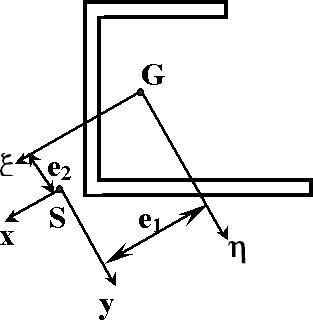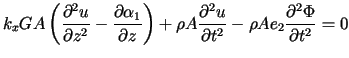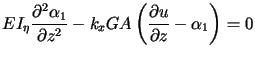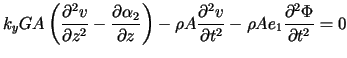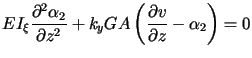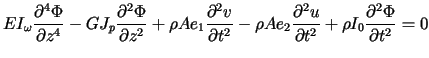where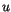and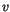are deflections of shear centre inanddirections respectively,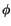is angle of rotation of cross-section,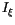and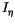are principal centroidal moments of inertia,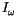is warping constant,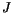is torsion constant,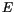and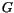are Young's and shear moduli respectively,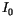is polar moment of inertia about shear centre,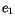and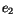are co-ordinates of centroid,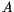is cross- sectional area,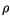is mass density,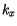and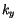are shear correction factors,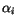are bending slopes and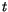is time.

Assuming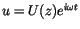,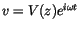,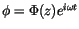and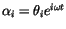five coupled equations are reduced to five independent equations by a procedure analogous to the solution of simultaneous algebraic equations where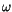is radian frequency. The result is an ordinary differential equation of 12 th order for each function. These latter equations are solved by taking a solution in the form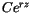.

#### Boundary Conditions

 Clamped end: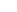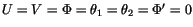Free end: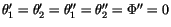;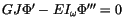Hinged end: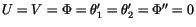#### Numerical evaluation and Conclusions

Numerical evaluation is performed for natural frequencies and the shear effect is discussed in detail. It is shown that the accuracy of the calculations of natural frequencies is considerably disturbed in higher modes when the shear effect is not taken account of.

References
1
J.M. Gere, "Torsional vibrations of beam of thin-walled open section", Journal of Applied Mechanics 21, 381 � 387, 1954.
2
Y. Yaman, "Vibration of open-section channels: a coupled flexural and torsional wave analysis", Journal of Sound and Vibration, 204 (1), 131-158, 1997. doi:10.1006/jsvi.1997.0933
3
A. Arpaci, S.E. Bozdag, E. Sunbuloglu, "Triply Coupled Vibrations of Thin- Walled Open Cross-Section Beams Including Rotary Inertia Effect", Journal of Sound and Vibration, in press, 2002. doi:10.1016/S0022-460X(02)00935-5

purchase the full-text of this paper (price £20)